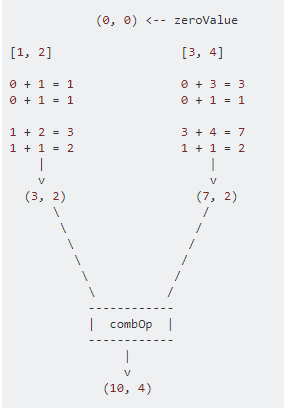2 views

I am looking for some better explanation of the aggregate functionality that is available via spark in python.

The example I have is as follows (using pyspark from Spark 1.2.0 version)

sc.parallelize([1,2,3,4]).aggregate(
(0, 0),
(lambda acc, value: (acc + value, acc + 1)),
(lambda acc1, acc2: (acc1 + acc2, acc1 + acc2)))

Output:

(10, 4)
I get the expected result (10,4) which is sum of 1+2+3+4 and 4 elements. If I change the initial value passed to the aggregate function to (1,0) from (0,0) I get the following result

sc.parallelize([1,2,3,4]).aggregate(
(1, 0),
(lambda acc, value: (acc + value, acc + 1)),
(lambda acc1, acc2: (acc1 + acc2, acc1 + acc2)))

Output:

(19, 4)
The value increases by 9. If I change it to (2,0), the value goes to (28,4) and so on.

Can someone explain to me how this value is calculated? I expected the value to go up by 1 not by 9, expected to see (11,4) instead I am seeing (19,4)

by (32.3k points)

Firstly, lets talk about the aggregate function and its parameters.

aggregate(zeroValue, seqOp, combOp)

aggregate() lets you take an RDD and generate a single value that is of a different type than what was stored in the original RDD.

Parameters:

zeroValue: The initialization value, for your result, in the desired format.

seqOp: The operation you want to apply to RDD records. Runs once for every record in a partition.

combOp: Defines how the resulted objects (one for every partition), gets combined.

Compute the sum of a list and the length of that list. Return the result in a pair of (sum, length).

In a Spark shell, I first created a list with 4 elements, with 2 partitions:

listRDD = sc.parallelize([1,2,3,4], 2)

then I defined my seqOp, combOp:

listRDD.aggregate( (0, 0), seqOp, combOp)

Out: (10, 4)

As you can see, I gave descriptive names to my variables, but let me explain it further:

The first partition has the sublist [1, 2]. We will apply the seqOp to each element of that list and this will produce a local result, a pair of (sum, length), that will reflect the result locally, only in that first partition.

So, let's start: local_result gets initialized to the zeroValue parameter we provided the aggregate() with, i.e. (0, 0) and list_element is the first element of the list, i.e. 1. As a result this is what happens:

0 + 1 = 1

0 + 1 = 1

Now, the local result is (1, 1), that means, that so far, for the 1st partition, after processing only the first element, the sum is 1 and the length 1. Notice, that local_result gets updated from (0, 0), to (1, 1).

1 + 2 = 3

1 + 1 = 2

and now the local result is (3, 2), which will be the final result from the 1st partition, since they are no other elements in the sublist of the 1st partition.

Doing the same for 2nd partition, we get (7, 2).

Now we apply the combOp to each local result, so that we can form, the final, global result, like this: (3,2) + (7,2) = (10, 4)So now if the zeroValue is not (0, 0), but (1, 0), one would expect to get (8 + 4, 2 + 2) = (12, 4), which doesn't explain what you experience. Even if we alter the number of partitions of my example, I won't be able to get that again.